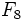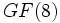# Field:F8

## Definition

This is the unique field (up to isomorphism) having eight elements. It is denoted$F_8$ or$GF(8)$. It can be defined as:$\! F_2[x]/(x^3 + x + 1)$.

where$F_2$ is the field of two elements.

## Related groups

Group functor Value GAP ID
additive group elementary abelian group:E8 (8,5)
multiplicative group cyclic group:Z7 (7,1)
general affine group of degree one general affine group:GA(1,8) (56,11)
projective special linear group of degree two projective special linear group:PSL(3,2) (168,42)

## GAP implementation

The field can be defined using GAP's GF function:

GF(8)